The SSM Procedure

Details

Subsections:

Throughout this section, vectors and matrices are denoted by boldface letters. Generally, Greek letters (such as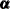,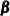, and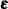) denote unobserved or latent quantities—often estimated from the data—that represent model parameters, latent states, or noise variables. Capital letters such as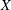,
, and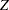are used to denote the observed data variables. Whenever there is no ambiguity, it is assumed that the matrices have appropriate dimensions when they are being multiplied—in particular, the vectors behave as column vectors or row vectors as the need arises. On many occasions, matrices are described inline—that is, they are described as parenthesized lists, in a rowwise fashion, with the rows separated by a comma. The term "dot product" is used to describe the scalar that results from the product of a row vector with a (conforming) column vector.